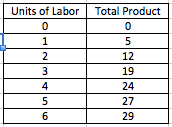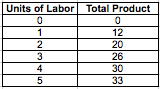# AP Microeconomics : Marginal Revenue Product of Labor MRP

## Example Questions

### Example Question #1 : Marginal Revenue Product Of Labor Mrp

The following question will refer to this table:If the wage paid to labor is $8 and the price of each unit of output is$2, how many units of labor will be hired?

one

four

two

five

three

four

Explanation:

A firm will hire labor until the marginal revenue product of labor is lower than the cost of labor/wage, which is $8. The marginal revenue product of labor is the marginal product of labor multiplied by the product's price. The marginal revenue of the fourth unit of labor is$10 (five units multiplied by $2) and the marginal revenue of the fifth unit of labor is$6 (three units multiplied by $2). Thus, the firm will hire four units of labor. ### Example Question #2 : Marginal Revenue Product Of Labor Mrp A firm is hiring labor in a perfectly competitive labor market. If the marginal revenue product of labor is greater than the wage rate, which of the following is true? Possible Answers: None of the other answers. The firm should invest in more capital. The firm is maximizing its profit. The firm should hire more labor. The firm is losing money. Correct answer: The firm should hire more labor. Explanation: A profit maximizing firm will hire labor until the marginal product of labor is greater than the wage rate. If the marginal product of labor is greater than the wage rate, then the firm should hire more labor until the two values are equal. ### Example Question #3 : Marginal Revenue Product Of Labor Mrp In a perfectly competitive labor market, an employer will continue hiring until ___________ . Possible Answers: wage equals marginal revenue product of labor. wage equals marginal cost of labor. wage equals marginal product of labor. wage equals marginal revenue. wage equals the price of the good or service produced. Correct answer: wage equals marginal revenue product of labor. Explanation: Like any market, the profit maximization level is the point at which marginal cost equals marginal revenue. In this case, the marginal cost of labor is the labor wage. Thus, the profit maximization point for the employer is the point at which wage equals the marginal revenue product of labor. ### Example Question #4 : Marginal Revenue Product Of Labor Mrp Refer to the following chart for this question:If each product is sold for$2, what is the marginal revenue product of labor for the fourth unit of labor?

$10$6

$12$4

$8 Correct answer:$8

Explanation:

The marginal revenue product of labor for the fourth unit of labor is its marginal product multiplied by the cost of the product. The marginal product of the fourth unit of labor is 4 (the difference between total production at four units of labor and three units of labor), and cost of the product is $2, so the marginal revenue product of labor for the fourth unit is$8.

### Example Question #5 : Marginal Revenue Product Of Labor Mrp

Refer to the following chart for this question:If each product is sold for $2, and each unit of labor costs$10, how many units of labor will the firm hire?

four

three

one

five

two